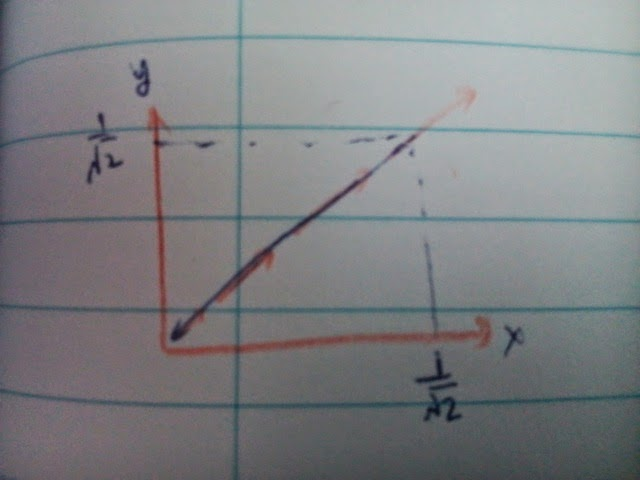## Vector field example 3Given a vector field \begin{align*} F &= \begin{pmatrix} y \\ x \end{pmatrix} \end{align*} an object move from $(1/\sqrt{2}, 1/\sqrt{2})$ to the origin, how much work is done?
Solution 1: \begin{align*} F\cdot dr &= F \cdot t \; ds \\ &= -|F|ds \\ &= -s \; ds \\ \end{align*} where $t$ is the tangent vector and $s$ is arc length of movement. You only need to integrate from 0 to 1 with respect to $s$, the answer should be $-1/2$
Solution 2: the normal way. Make use of the relation $y = x$.